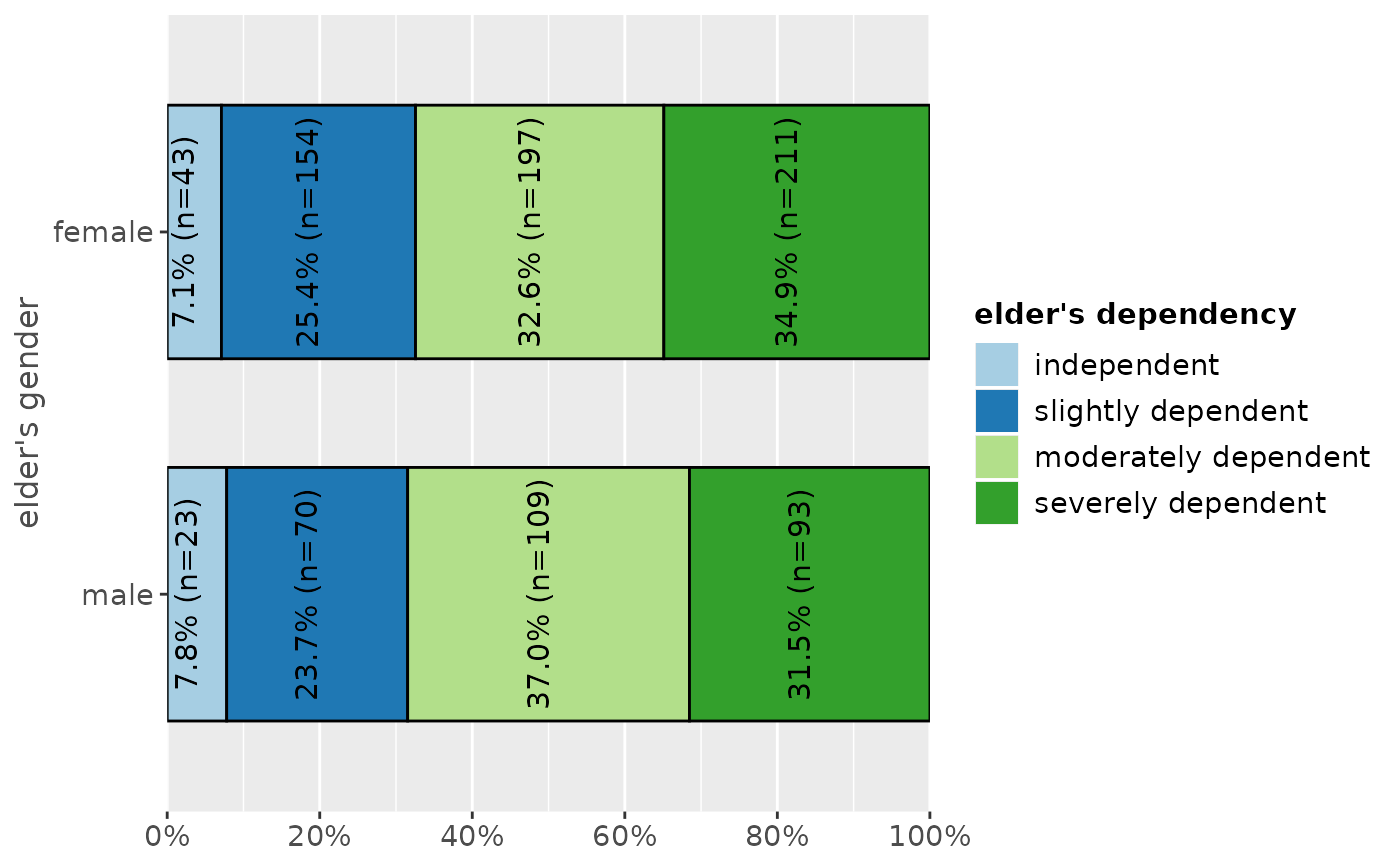Plot proportional crosstables (contingency tables) of two variables as ggplot diagram.

plot_xtab(
x,
grp,
type = c("bar", "line"),
margin = c("col", "cell", "row"),
bar.pos = c("dodge", "stack"),
title = "",
title.wtd.suffix = NULL,
axis.titles = NULL,
axis.labels = NULL,
legend.title = NULL,
legend.labels = NULL,
weight.by = NULL,
rev.order = FALSE,
show.values = TRUE,
show.n = TRUE,
show.prc = TRUE,
show.total = TRUE,
show.legend = TRUE,
show.summary = FALSE,
summary.pos = "r",
drop.empty = TRUE,
string.total = "Total",
wrap.title = 50,
wrap.labels = 15,
wrap.legend.title = 20,
wrap.legend.labels = 20,
geom.size = 0.7,
geom.spacing = 0.1,
geom.colors = "Paired",
dot.size = 3,
smooth.lines = FALSE,
grid.breaks = 0.2,
expand.grid = FALSE,
ylim = NULL,
vjust = "bottom",
hjust = "center",
y.offset = NULL,
coord.flip = FALSE
)

## Arguments

x

A vector of values (variable) describing the bars which make up the plot.

grp

Grouping variable of same length as x, where x is grouped into the categories represented by grp.

type

Plot type. may be either "bar" (default) for bar charts, or "line" for line diagram.

margin

Indicates which data of the proportional table should be plotted. Use "row" for calculating row percentages, "col" for column percentages and "cell" for cell percentages. If margin = "col", an additional bar with the total sum of each column can be added to the plot (see show.total).

bar.pos

Indicates whether bars should be positioned side-by-side (default), or stacked (bar.pos = "stack"). May be abbreviated.

title

character vector, used as plot title. Depending on plot type and function, will be set automatically. If title = "", no title is printed. For effect-plots, may also be a character vector of length > 1, to define titles for each sub-plot or facet.

title.wtd.suffix

Suffix (as string) for the title, if weight.by is specified, e.g. title.wtd.suffix=" (weighted)". Default is NULL, so title will not have a suffix when cases are weighted.

axis.titles

character vector of length one or two, defining the title(s) for the x-axis and y-axis.

axis.labels

character vector with labels used as axis labels. Optional argument, since in most cases, axis labels are set automatically.

legend.title

character vector, used as title for the plot legend.

legend.labels

character vector with labels for the guide/legend.

weight.by

Vector of weights that will be applied to weight all cases. Must be a vector of same length as the input vector. Default is NULL, so no weights are used.

rev.order

Logical, if TRUE, order of categories (groups) is reversed.

show.values

Logical, whether values should be plotted or not.

show.n

logical, if TRUE, adds total number of cases for each group or category to the labels.

show.prc

logical, if TRUE (default), percentage values are plotted to each bar If FALSE, percentage values are removed.

show.total

When margin = "col", an additional bar with the sum within each category and it's percentages will be added to each category.

show.legend

logical, if TRUE, and depending on plot type and function, a legend is added to the plot.

show.summary

logical, if TRUE (default), a summary with chi-squared statistics (see chisq.test), Cramer's V or Phi-value etc. is shown. If a cell contains expected values lower than five (or lower than 10 if df is 1), the Fisher's exact test (see fisher.test) is computed instead of chi-squared test. If the table's matrix is larger than 2x2, Fisher's exact test with Monte Carlo simulation is computed.

summary.pos

position of the model summary which is printed when show.summary is TRUE. Default is "r", i.e. it's printed to the upper right corner. Use "l" for upper left corner.

drop.empty

Logical, if TRUE and the variable's values are labeled, values that have no observations are still printed in the table (with frequency 0). If FALSE, values / factor levels with no occurrence in the data are omitted from the output.

string.total

String for the legend label when a total-column is added. Only applies if show.total = TRUE. Default is "Total".

wrap.title

numeric, determines how many chars of the plot title are displayed in one line and when a line break is inserted.

wrap.labels

numeric, determines how many chars of the value, variable or axis labels are displayed in one line and when a line break is inserted.

wrap.legend.title

numeric, determines how many chars of the legend's title are displayed in one line and when a line break is inserted.

wrap.legend.labels

numeric, determines how many chars of the legend labels are displayed in one line and when a line break is inserted.

geom.size

size resp. width of the geoms (bar width, line thickness or point size, depending on plot type and function). Note that bar and bin widths mostly need smaller values than dot sizes.

geom.spacing

the spacing between geoms (i.e. bar spacing)

geom.colors

user defined color for geoms. See 'Details' in plot_grpfrq.

dot.size

Dot size, only applies, when argument type = "line".

smooth.lines

prints a smooth line curve. Only applies, when argument type = "line".

grid.breaks

numeric; sets the distance between breaks for the axis, i.e. at every grid.breaks'th position a major grid is being printed.

expand.grid

logical, if TRUE, the plot grid is expanded, i.e. there is a small margin between axes and plotting region. Default is FALSE.

ylim

numeric vector of length two, defining lower and upper axis limits of the y scale. By default, this argument is set to NULL, i.e. the y-axis fits to the required range of the data.

vjust

character vector, indicating the vertical position of value labels. Allowed are same values as for vjust aesthetics from ggplot2: "left", "center", "right", "bottom", "middle", "top" and new options like "inward" and "outward", which align text towards and away from the center of the plot respectively.

hjust

character vector, indicating the horizontal position of value labels. Allowed are same values as for vjust aesthetics from ggplot2: "left", "center", "right", "bottom", "middle", "top" and new options like "inward" and "outward", which align text towards and away from the center of the plot respectively.

y.offset

numeric, offset for text labels when their alignment is adjusted to the top/bottom of the geom (see hjust and vjust).

coord.flip

logical, if TRUE, the x and y axis are swapped.

A ggplot-object.

## Examples

# create 4-category-items
grp <- sample(1:4, 100, replace = TRUE)
# create 3-category-items
x <- sample(1:3, 100, replace = TRUE)

# plot "cross tablulation" of x and grp
plot_xtab(x, grp)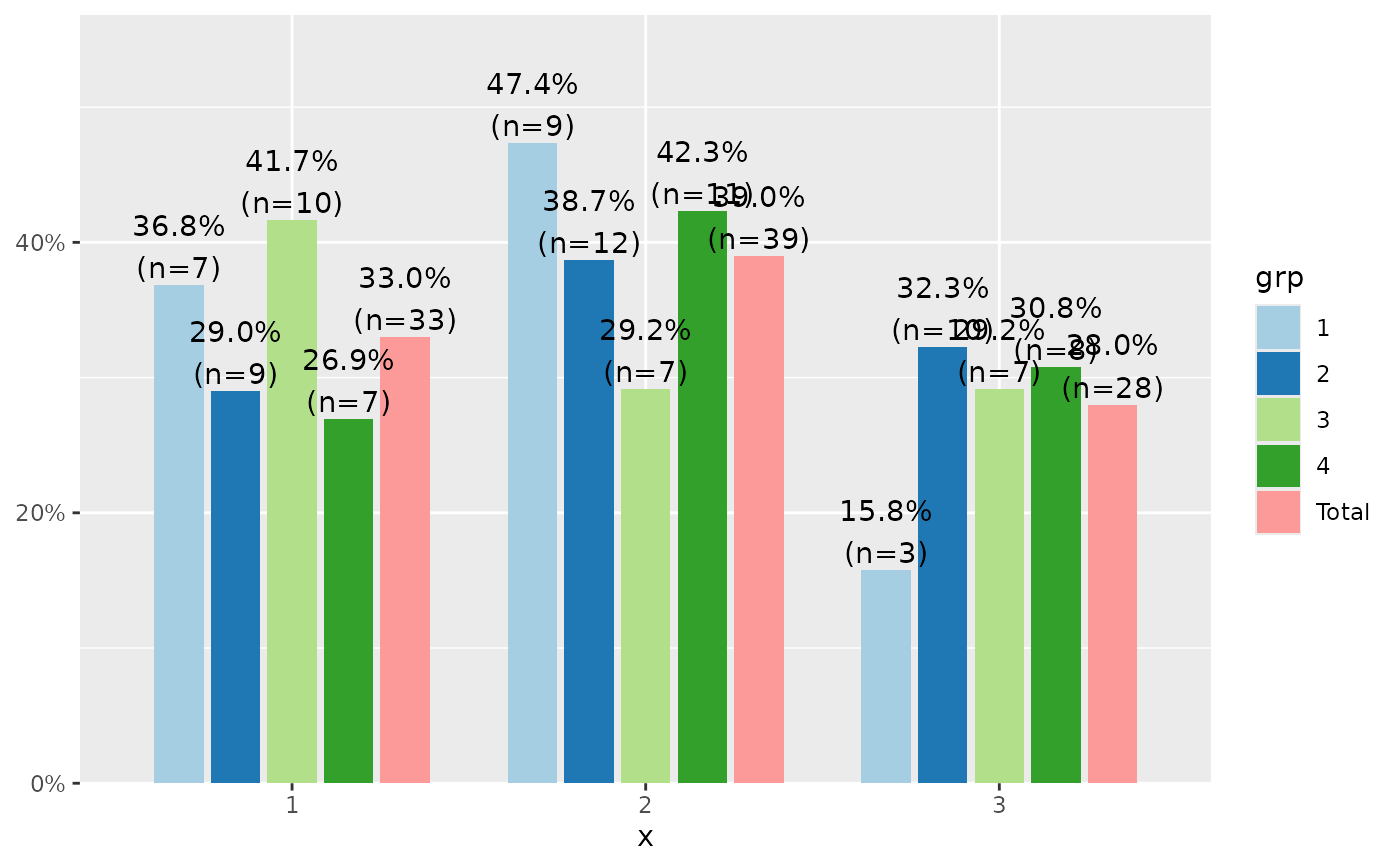# plot "cross tablulation" of x and y, including labels
plot_xtab(x, grp, axis.labels = c("low", "mid", "high"),
legend.labels = c("Grp 1", "Grp 2", "Grp 3", "Grp 4"))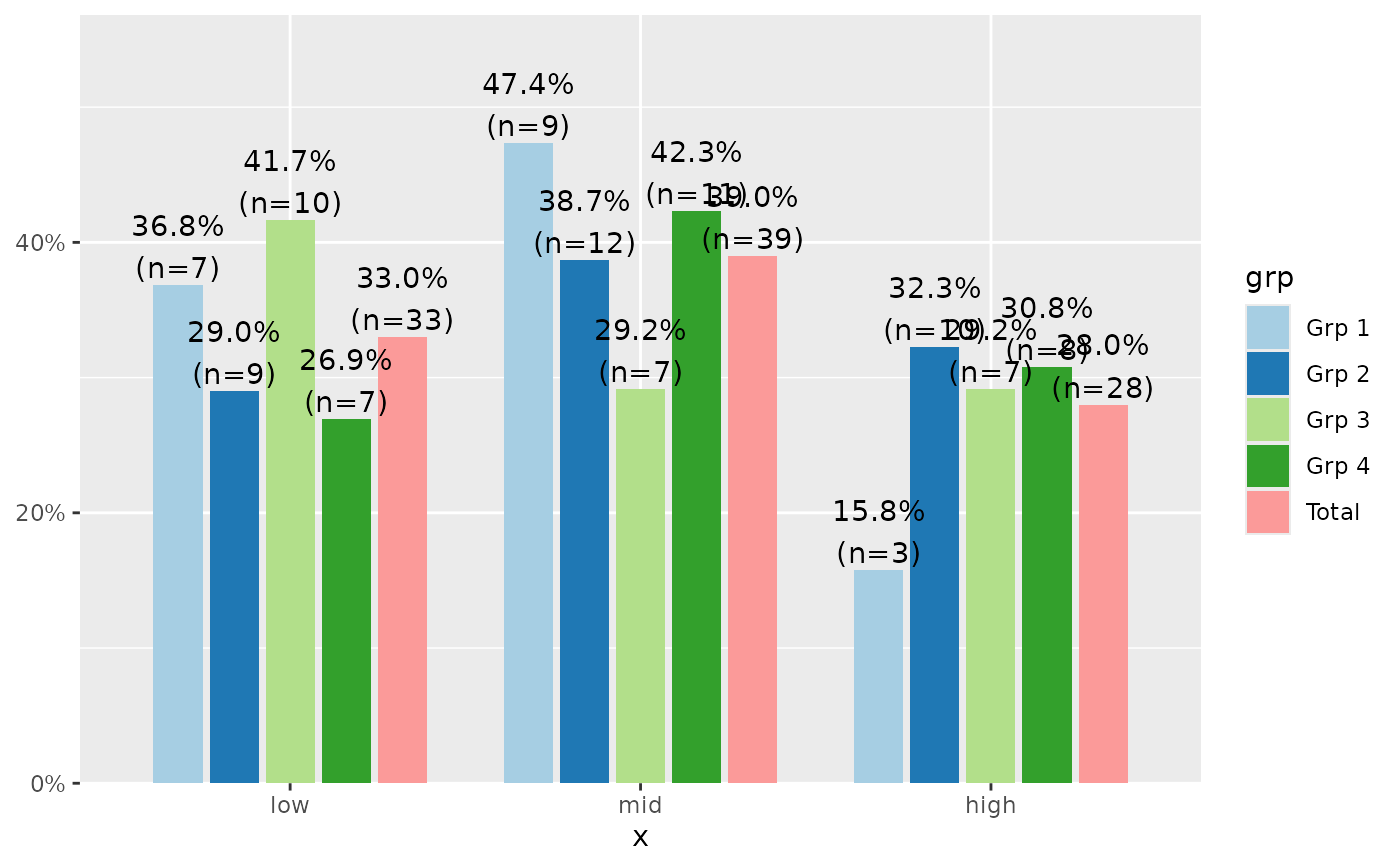# plot "cross tablulation" of x and grp
# as stacked proportional bars
plot_xtab(x, grp, margin = "row", bar.pos = "stack",
show.summary = TRUE, coord.flip = TRUE)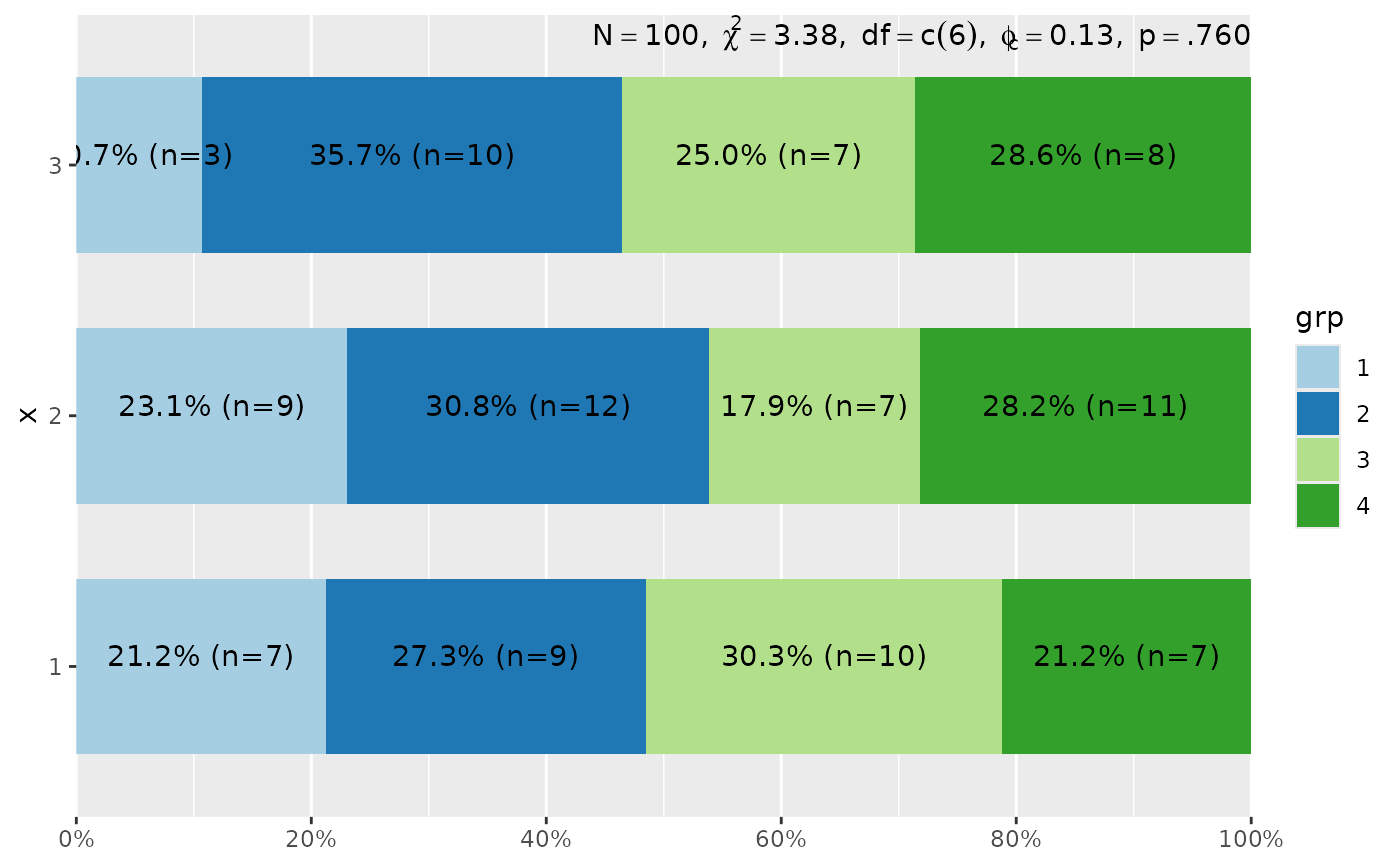# example with vertical labels
library(sjmisc)
library(sjlabelled)
data(efc)
set_theme(geom.label.angle = 90)
plot_xtab(efc$e42dep, efc$e16sex, vjust = "center", hjust = "bottom")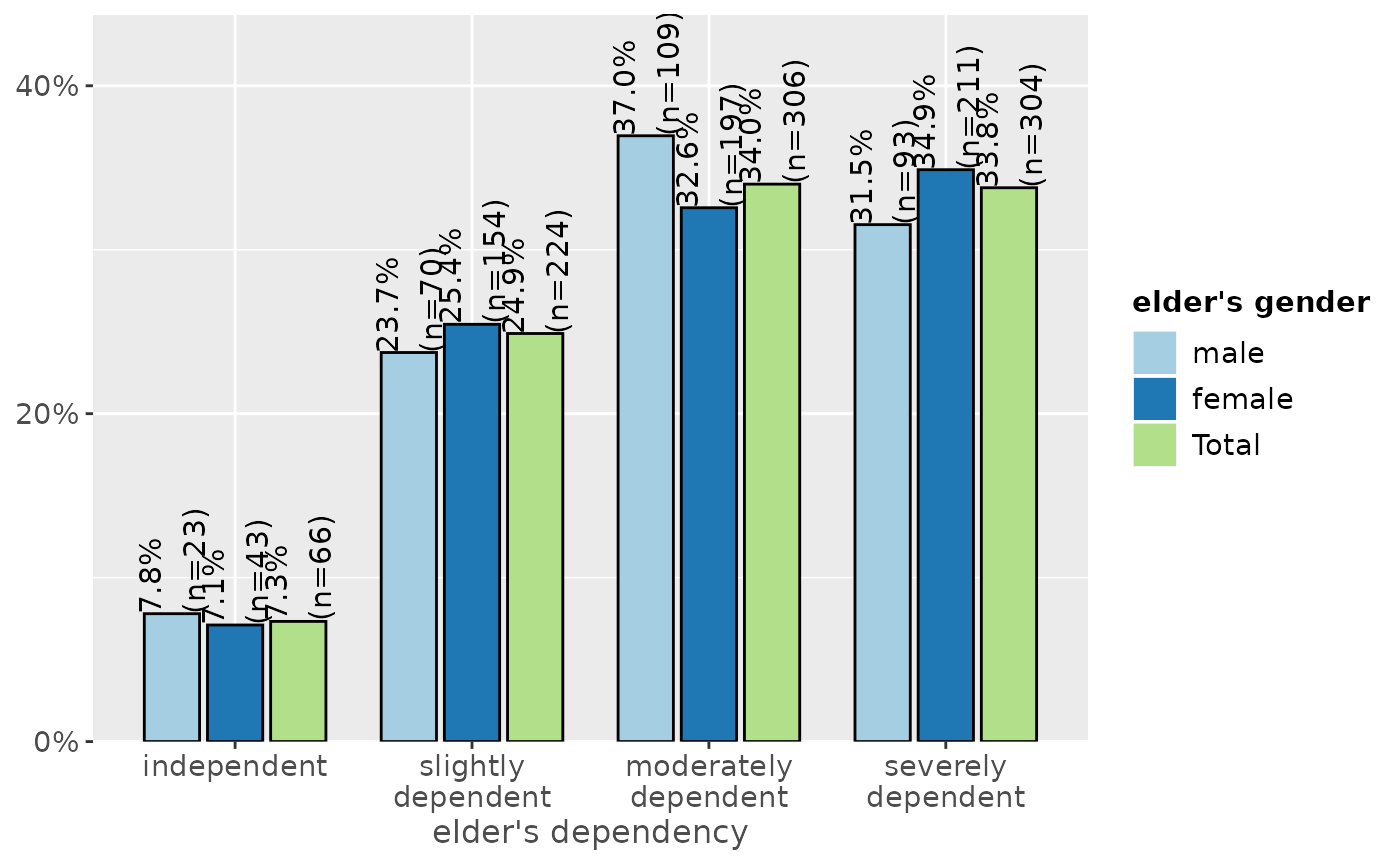# grouped bars with EUROFAMCARE sample dataset
# dataset was importet from an SPSS-file,
data(efc)
efc.val <- get_labels(efc)
efc.var <- get_label(efc)

plot_xtab(efc$e42dep, efc$e16sex, title = efc.var['e42dep'],
axis.labels = efc.val[['e42dep']], legend.title = efc.var['e16sex'],
legend.labels = efc.val[['e16sex']])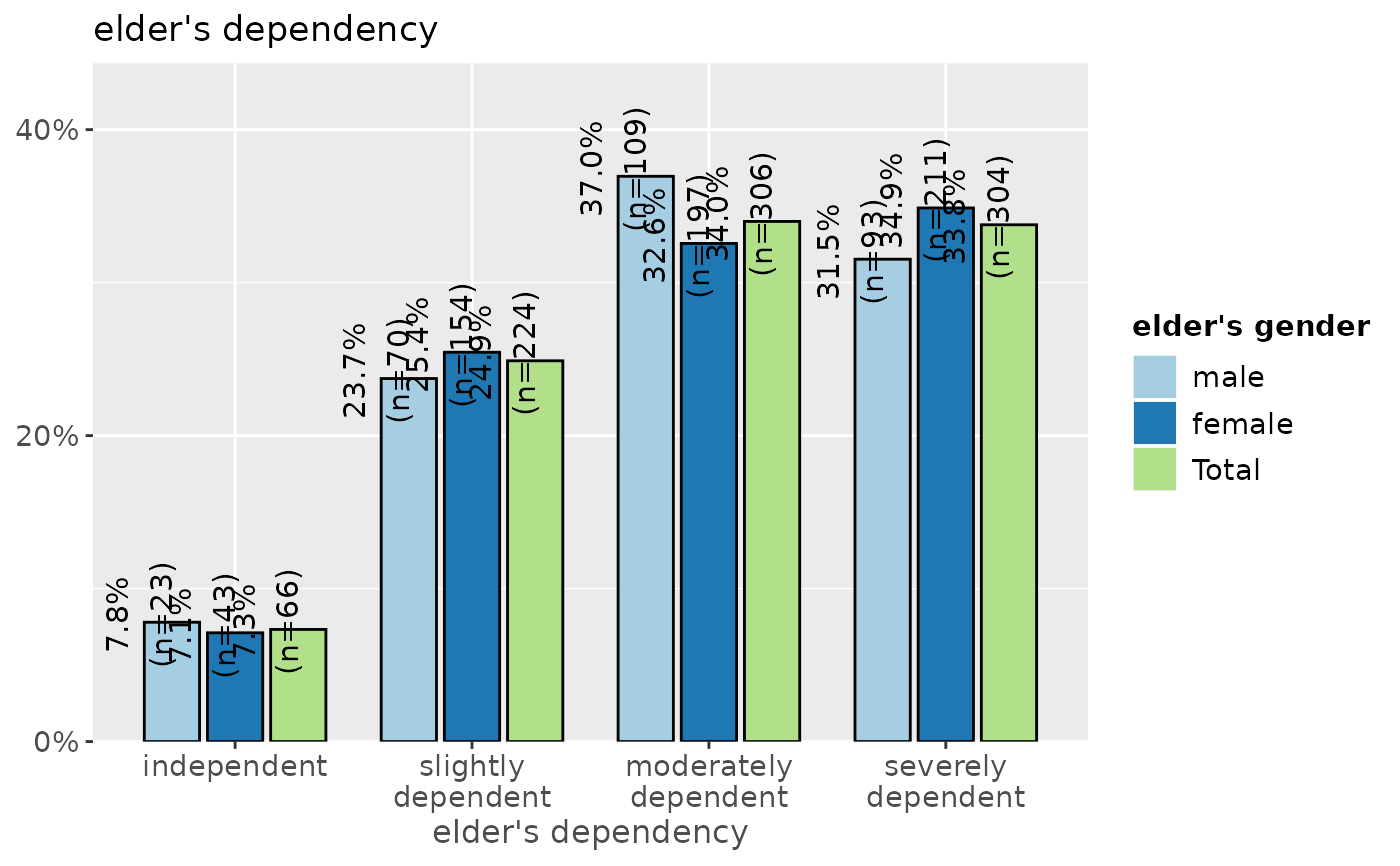plot_xtab(efc$e16sex, efc$e42dep, title = efc.var['e16sex'],
axis.labels = efc.val[['e16sex']], legend.title = efc.var['e42dep'],
legend.labels = efc.val[['e42dep']])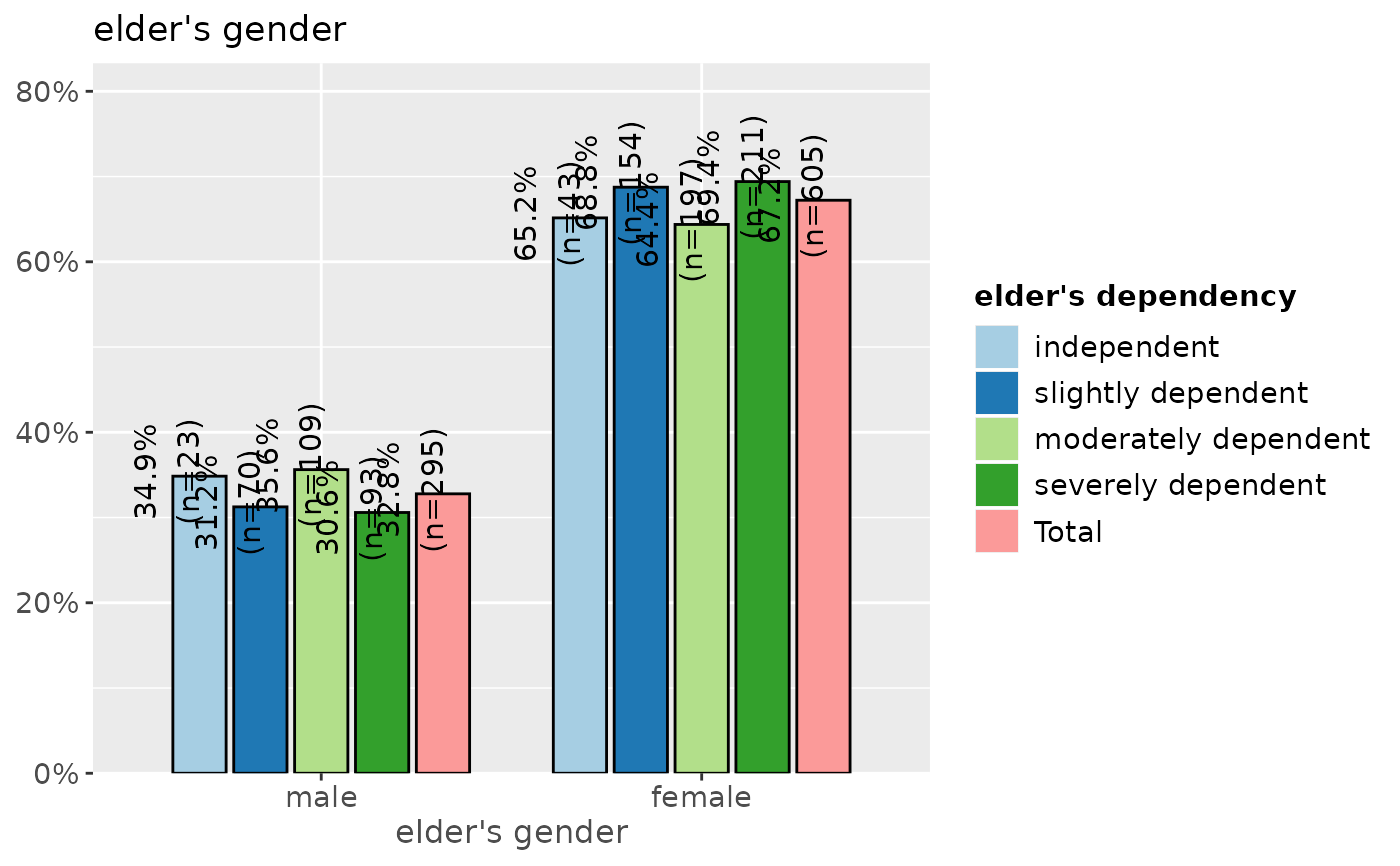# -------------------------------
# auto-detection of labels works here
# so no need to specify labels. For
# title-auto-detection, use NULL
# -------------------------------
plot_xtab(efc$e16sex, efc$e42dep, title = NULL)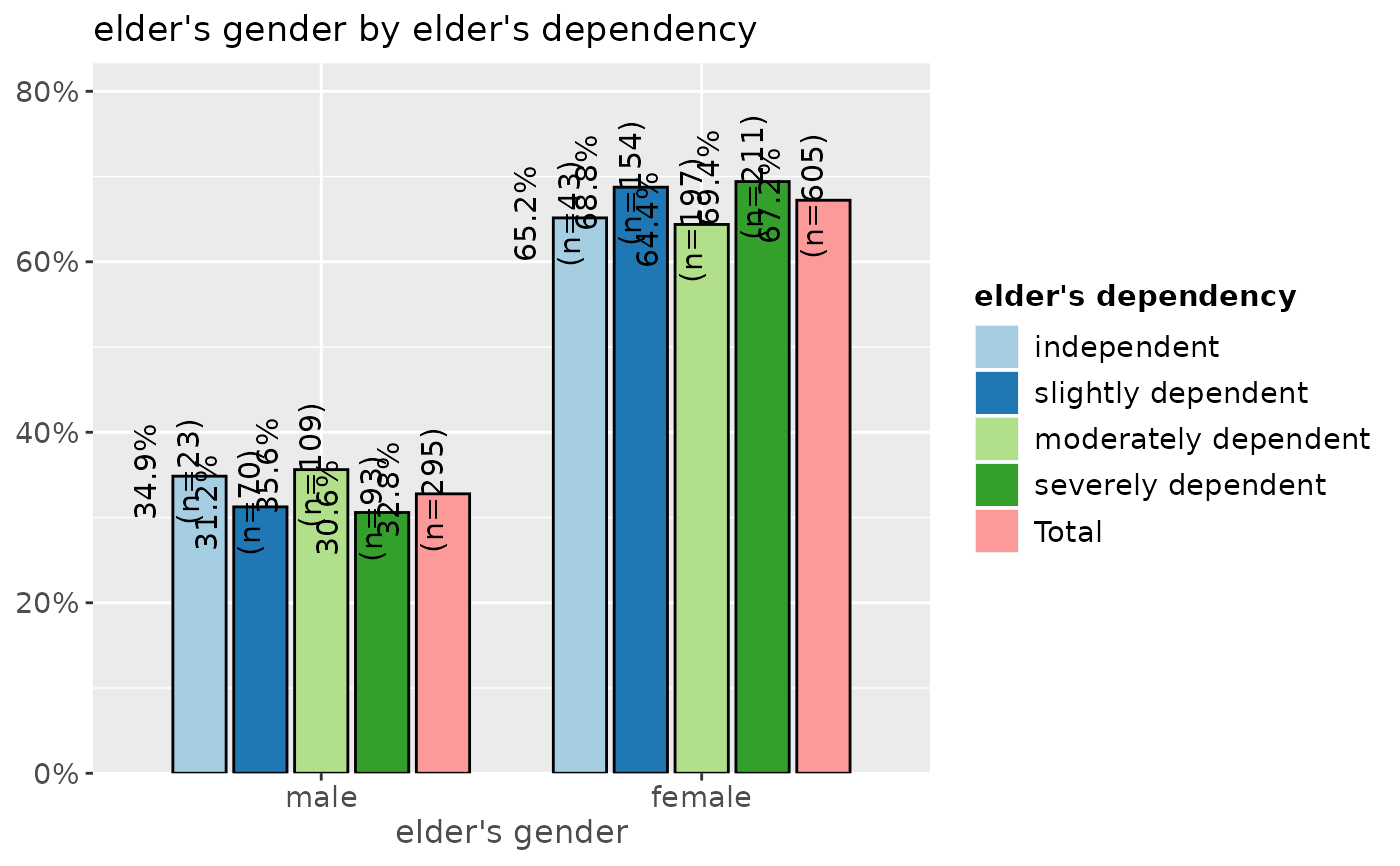plot_xtab(efc$e16sex, efc$e42dep, margin = "row",
bar.pos = "stack", coord.flip = TRUE)